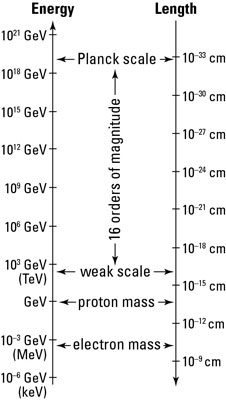## The Standard Model of Physics

Our intuition as humans tells us that there is a reason why everything is the way it is. If something is different or looks a bit out of place we encounter a strong desire to work out the reason behind this discrepancy. The history of physics could be seen as the story of how we find discrepancies between the way nature behaves and the way we expect it to. Once we find these discrepancies, the job of physics is to explain why they are there.

This is the situation that physics is in today. In particle physics we have one of the most accurate and successful theories ever – the Standard Model – but there are many things about it that seem “out of place”. Here’s a picture summarising the structure of the Standard Model:

This doesn’t look appealing at all – it looks like a total mess! We have a bunch of different particles with seemingly arbitrary properties. It doesn’t look elegant enough to be a fundamental theory at all.

## Naturalness

Now we must be careful when we make arguments based on elegance in physics. Arguments such as these are known as naturalness arguments. There is no reason of course for nature to appear elegant at the fundamental level, so it would be wrong to mark a theory down based on its inelegance.

Fortunately we know that the Standard Model cannot possibly be all there is to fundamental physics because it leaves out one of the four fundamental forces entirely – gravity. There is no account of gravitational interactions in the Standard Model, so there is no way it can be a theory of everything.

## The Hierarchy Problem

There is an additional problem with the standard model to do with its inelegance called the Hierarchy Problem. This is an unsolved problem in Physics which may or may not be to do with the lack of gravity in the model. The Hierarchy Problem looks at the different energy scales that physics occurs on. The strongest force is the aptly named “strong force”, while the weakest force in the Standard model is the also appropriately named “weak force”.

We can compare the strengths of the forces in the Standard Model to the strength of the gravitational force and we find that there is a huge discrepancy between their relative strengths. The Standard Model tells us that the weak force is actually$10^{24}$ times stronger than the gravitational force.

To get a feel of how big this discrepancy is, if the strength of the gravitational force is equivalent to getting hit with a few apples, the weak force is equivalent to getting hit with the entire Earth.The difference in energy scales between the weak force and gravity

## Supersymmetry

So the relative strengths of forces seem very unnatural, they operate on ridiculously different energy scales. The natural resolution to this problem is to conjecture that there is some new, undiscovered physics that lies in between these two massive energy scales. This new physics is called Supersymmetry.

What supersymmetry proposes is that there is an additional kind of symmetry in nature. We have familiar symmetries in physics such as translational and rotational symmetry: it makes no difference to physics if you move your entire system a metre to the left, or rotate the entire system. Supersymmetry proposes that there are additional symmetries in nature between various different particles. Fundamental particles in the Standard Model are split into two groups: fermions and bosons. Supersymmetry proposes that every fermion has a corresponding boson which it is related to by a supersymmetric transformation (kind of like a rotation, but in an abstract “superspace” not normal space).

While supersymmetry is a much more elegant theory of fundamental physics than the standard model, there is currently no experimental evidence that it actually exists in nature.

## Supersymmetry and the Hierarchy Problem

So how does supersymmetry solve the Hierarchy Problem? A large part of the hierarchy problem comes down to quantum corrections. This is a common theme in theoretical physics – we formulate our theories in a classical way and then add “quantum corrections” to them to take into account quantum theory.

In the Standard model we get a huge correction to what’s called the vacuum expectation value of the Higgs boson from its interactions with the top quark. (The vacuum expectation value is basically related to the energy of the Higgs field). This is a particular manifestation of the Hierarchy Problem. The correction to the Higgs means that its energy scale is comparable to that of the gravitational (or Planck) scale. But if this is the case why is it so much larger than the weak scale?

It is here that supersymmetry proposes a resolution. If we take into account supersymmetry, then we get an additional contribution to the Higgs field from the superpartner of the top quark. Recall that in supersymmetry, every fermion has a boson which it is related to supersymmetrically. The top quark is a fermion and so is related to an as of yet undiscovered boson, which will also contribute to the Higgs field.

The magic of supersymmetry is that the quantum corrections to the Higgs field from the superpartner particles exactly cancel out the corrections from the particles we know about already. This would mean that the Higgs energy scale is not ridiculously large compared to the weak scale, and this Hierarchy problem vanishes.

Unfortunately the current lack of evidence for supersymmetry at the LHC means that it might be the case that nature is not supersymmetric. Even if we do rule out supersymmetry however, the Hierarchy problem will still remain and will be become more mysterious than ever.